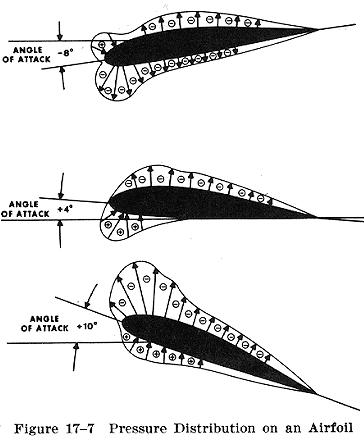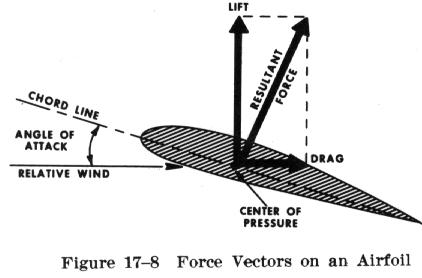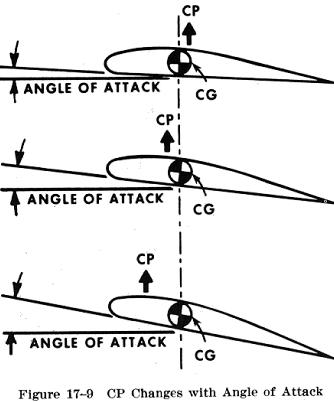PressureDistribution Pressure Distribution

 From experiments conducted on wind tunnel models and on full size airplanes, it has been determined that as air flows along the surface of a wing at different angles of attack there are regions along the surface where the pressure is negative, or less than atmospheric, and regions where the pressure is positive, or greater than atmospheric. This negative pressure on the upper surface creates a relatively larger force on the wing than is caused by the positive pressure resulting from the air striking the lower wing surface. Figure 17-7 shows the pressure distribution along an airfoil at three different angles of attack. In general, at high angles of attack the center of pressure moves forward, while at low angles of attack the center of pressure moves aft. In the design of wing structures this center of pressure travel is very important, since it affects the position of the airloads imposed on the wing structure in low angle of attack conditions and high angle of attack conditions. The airplane's aerodynamic balance and controllability are governed by changes in the center of pressure. The center of pressure is determined through calculation and wind tunnel tests by varying the airfoil's angle of attack through normal operating extremes. As the angle of attack is changed, so are the various pressure distribution characteristics (Fig. 17-7). Positive (+) and negative (-) pressure forces are totaled for each angle of attack and the resultant force is obtained. The total resultant pressure isrepresented by the resultant force vector shown in Fig. 17-8. The point of application of this force vector is termed the "center of pressure" (CP). For any given angle of attack, the center of pressure is the point where the resultant force crosses the chord line. This point is expressed as a percentage of the chord of the airfoil. A center of pressure at 30 percent of a 60 inch chord would be 18 inches aft of the wing's leading edge. It would appear then that if the designer would place the wing so that its center of pressure was at the airplane's center of gravity, the airplane would always balance. The difficulty arises, however, that the location of the center of pressure changes with change in the airfoil's angle of attack (Fig. 17-9).In the airplane's normal range of flight attitudes, if the angle of attack is increased, the center of pressure moves forward; and if decreased, it moves rearward. Since the center of gravity is fixed at one point, it is evident that as the angle of attack increases, the center of lift (CP) moves ahead of the center of gravity, creating a force which tends to raise the nose of the airplane or tends to increase the angle of attack still more. On the other hand, if the angle of attack is decreased, the center of lift (CP) moves aft and tends to decrease the angle a greater amount. It is seen then, that the ordinary airfoil is inherently unstable, and that an auxiliary device, such as the horizontal tail surface, must be added to make the airplane balance longitudinally. The balancing forces imparted by the tail surfaces will be discussed later in the chapter.
 The balance of an airplane in flight depends, therefore, on the relative position of the center of gravity (CG) and the center of pressure (CP) of the airfoil. Experience has shown that an airplane with the center of gravity in the vicinity of 20 percent of the wing chord can be made to balance and fly satisfactorily.    The tapered wing presents a variety of wing chords throughout the span of the wing. It becomes necessary then, to specify some chord about which the point of balance can be expressed. This chord, known as the mean aerodynamic chord (MAC), usually is defined as the chord of an imaginary untapered wing which would have the same center of pressure characteristics as the wing in question.    Airplane loading and weight distribution also affect center of gravity and cause additional forces which in turn affect airplane balance. This will be discussed in a later section.